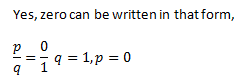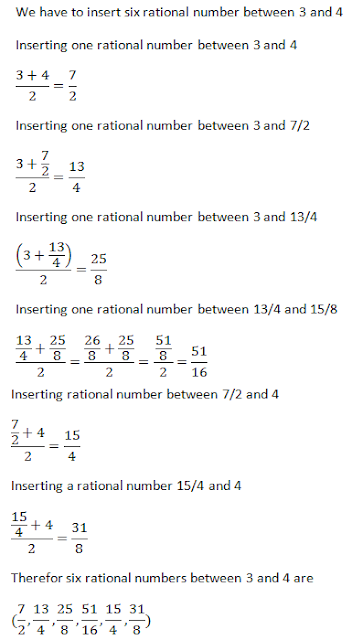#### Chapter 1 Number System R.D. Sharma Solutions for Class 9th Math Exercise 1.1

Exercise 1.1

Level 1

1. Is zero rational number ? Can you write it in the form p/q , where p and q are integers and q are integers and q ≠ 0 ?

Solution2. Find five rational numbers between 1 and 2.

Solution3. Find six rational numbers between 3 and 4.

Solution4. Find five rational numbers between 3/5 and 4/5

SolutionIn order to find number of rational number between two fraction, multiply both numerator and denominator by any integer greater than the number of rational number you have to find.
For example in this case, if we have to find 5 numbers between two fractions of same denominator, multiply both denominator and numerator by any number more than 5, may be 6.

5. Are the following statements true or false? Give reasons for you answer.
(i) Every whole number is a rational number.
(ii) Every integer is a rational number.
(iii) Every rational number is an integer.
(iv) Every natural number is a whole number.
(v) Every integer is a whole number.
(vi) Every rational number is a whole number.

Solution

(i) False
Zero is a whole number but not  a natural number.

(ii) True
Every integer can be expressed in the form of p/q,  p and q  are integer, qis not zero, for example
-4 is integer can be written as -4/1 -16/4, 20/-5 etc and 5 an integer can be written as 5/1, 25/5, -10/-2 etc

(iii) False
7/5. -6/4 etc are rational number but they are not integer.

(iv ) True
Natural numbers are all counting numbers and Whole number is 0 and counting numbers.

(v) False